#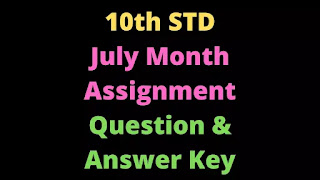10th July Month Assignment Answer Key

10th Standard Maths July Month Assignment 2 Question Paper and Answer key Download PDF. 10thh Maths Unit 2 Numbers and Sequence TN 10th All Subject July Month Assignment 2 Answers, 10th Tamil, English, Maths, Science, Social Science July Month Assignment Answer key Tamil Medium, English Medium Download PDF. TN SCERT,  ASSIGNMENT 1 QUESTION & ANSWER KEY TN SCERT

• Class: 10
• Subject : Maths
• Unit:  2 Numbers and Sequence

### We get 412 = 340 × 1 + 72The remainder 72 ≠ 0Again applying Euclid’s division algorithm340 = 72 × 4 + 52The remainder 52 ≠ 0.Again applying Euclid’s division algorithm72 = 52 × 1 + 20The remainder 20 ≠ 0.Again applying Euclid’s division algorithm,52 = 20 × 2 + 12The remainder 12 ≠ 0.Again applying Euclid’s division algorithm.20 = 12 × 1 + 8The remainder 8 ≠ 0.Again applying Euclid’s division algorithm12 = 8 × 1 + 4The remainder 4 ≠ 0.Again applying Euclid’s division algorithm8 = 4 × 2 + 0The remainder is zero.Therefore H.C.F. of 340 and 412 is 4.   12. If 13824 = 2a x 3b, then find the value of a and b. Using factor tree method factorisee 1382413. Solve 8x = 1 ( mod 11)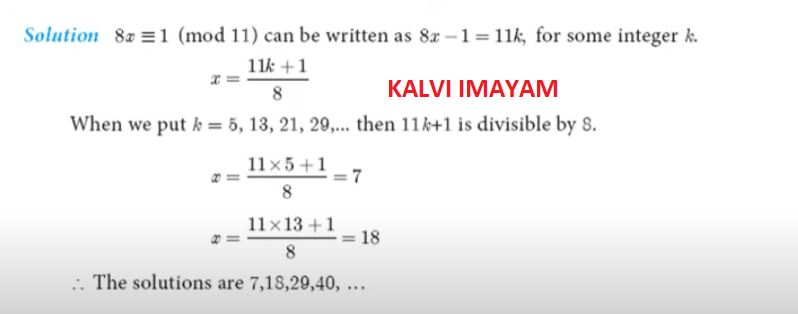14. Given that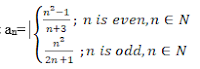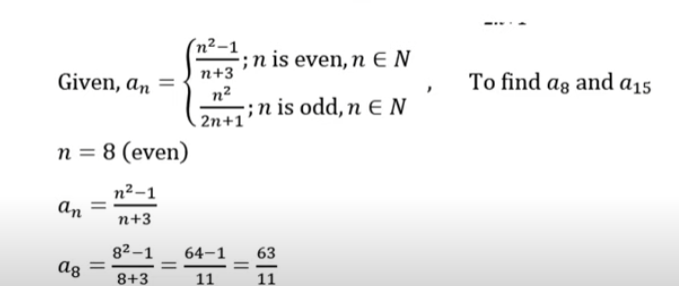15. Which term of an A.P 16, 11, 6, 1…is -54? Solution: A.P = 16, 11,6, 1, ……….. It is given that tn = -54 a = 16,  d = t2 – t1     = 11 – 16    d  = -5 ∴ tn = a + (n – 1)d -54 = 16 + (n – 1) (-5) -54 = 16 – 5n + 5 21 – 5n = -54 -5n = -54 -21 -5n = -75 n = $$\frac { 75 }{ 5 }$$ =15 ∴ 15th term is -54. 16. Find the sum of first six terms of GP 5, 15, 45 …17. If 1+2+3+…+n= 666 then find n.####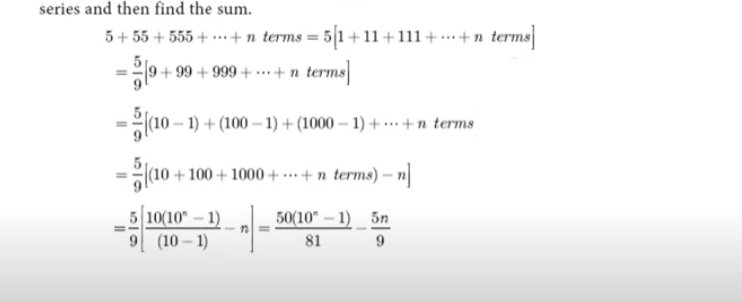19.Find the HCF of 396, 504, 636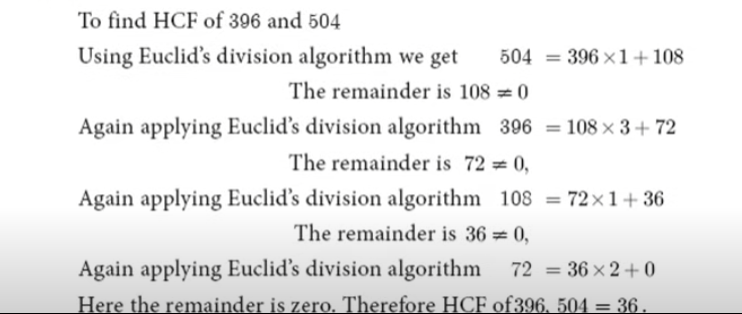#### 20.In an AP, the sum of four consecutive terms is 28 and the sum of their squares is 276. Find that four numbers.#### 21.Rekha has 15 square colour papers of size 10cm, 11 cm, 12cm …. 24cm. How much area can be decorated with these colour papers?